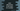# How to add zeros to the start of a number in Java## Java add zeros to the start of a number :

In this Java tutorial, we will learn how to modify a number by padding zeros at start. For example, if the number is 123 and if we want to add three zeros to the start of this number, it will become 000123. We will show you two different ways to achieve this in this tutorial :

### Using String.format() :

String class has one static method format that can be used to format an integer number to string with leading padded zeros. For example, if we want to add 3 zeros to the number 456, we can use format() as below :

``````class Example {
public static void main(String args[]) {
int givenNumber = 456;
String formattedNumber = String.format("%06d", givenNumber);
System.out.println("Formatted number is : " + formattedNumber);
}
}``````

It will give you the below output :

``Formatted number is : 000456``

As you can see that we have used %06d in the String.format() because we already have 3 digits in the number and to add 3 more zeros, we need total 6 digits. So, 06.

This program can be extended to get the input from the user. The user will provide the number and also the zero count and the program will simply print out the output.

``````import java.util.Scanner;

class Example {
public static void main(String args[]) {
//1
String givenNumber;
int zeroCount;

//2
Scanner sc = new Scanner(System.in);

//3
System.out.println("Enter a number : ");
givenNumber = sc.next();

//4
System.out.println("Enter total numbers of zeros : ");
zeroCount = sc.nextInt();

//5
String formatter = "%0" + (givenNumber.length() + zeroCount) + "d";

//6
System.out.println("Final number : " + String.format(formatter, Integer.valueOf(givenNumber)));
}
}``````

### Sample output :

``````Enter a number :
123
Enter total numbers of zeros :
3
Final number : 000123

Enter a number :
1
Enter total numbers of zeros :
4
Final number : 00001

34
Enter total numbers of zeros :
1
Final number : 034``````

### Explanation :

The commented numbers in the above program denote the step numbers below :

1. Create two variables. One string variable to store the user input number and one integer variable to store the count of zeros.
2. Create one Scanner object to read the user input values.
3. Ask the user to enter a number. Read it and store it in the string variable.
4. Ask the user to enter the total number of zero to add to the left of the above number. Read it and store it in the integer variable.
5. Now, we need to create the formatter that we are going to use in the String.format() method. If the number contains 2 digits and we want to add 3 zeros to the left of it, the formatter will be %02d. Create it by calculating the size of the number and the count of zeroes we have collected in the above steps.
6. Finally, print the number to the user using String.format().

### Using DecimalFormat :

Similar to String.format(), we can also use DecimalFormat to add zeroes to the front of a number. First, we need to create one DecimalFormat object with a specific format and then we can call format() method on this object to get the specific result.

Example :

``````import java.text.DecimalFormat;
import java.util.Scanner;

class Example {
public static void main(String args[]) {
DecimalFormat df = new DecimalFormat("00000");
int number = 245;

System.out.println("Final number : " + df.format(number));
}
}``````

It will print 00245 as output. Note that .format returns one string.

Instead of hardcoding the 0 while creating a DecimalFormat object, we can also create it dynamically. Let’s create one example like we have did for String.format() above :

``````import java.text.DecimalFormat;
import java.util.Arrays;
import java.util.Scanner;

class Example {
public static void main(String args[]) {
int givenNumber;
int zeroCount;

Scanner sc = new Scanner(System.in);

System.out.println("Enter a number : ");
givenNumber = sc.nextInt();

System.out.println("Enter total numbers of zeros : ");
zeroCount = sc.nextInt();

char[] zerosArray = new char[zeroCount + String.valueOf(givenNumber).length()];
Arrays.fill(zerosArray, '0');

String formatter = String.valueOf(zerosArray);

DecimalFormat df = new DecimalFormat(formatter);

System.out.println("Final number : " + df.format(givenNumber));
}
}``````

#### Sample output :

``````Enter a number :
456
Enter total numbers of zeros :
3
Final number : 000456

Enter a number :
3
Enter total numbers of zeros :
5
Final number : 000003

Enter a number :
45
Enter total numbers of zeros :
1
Final number : 045``````

As you can see that the main step in this program is while creating the array zerosArray. The size of the array is sum of total number of digits in the given number + number of zeros. That’s it.

### Conclusion :

We have seen two different ways to add zeros to the start of a number string in Java.We have also learn how to implement it dynamically. Go through the examples above and drop one comment below if you have any queries.